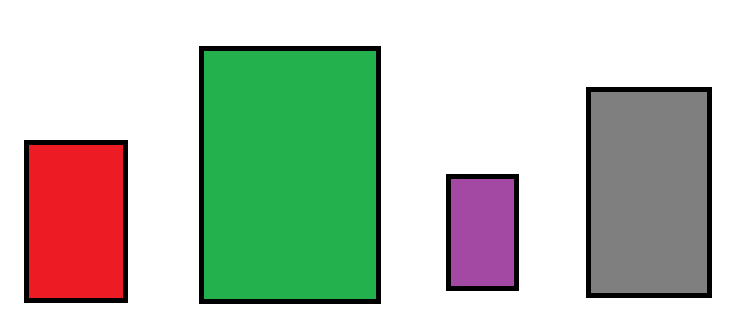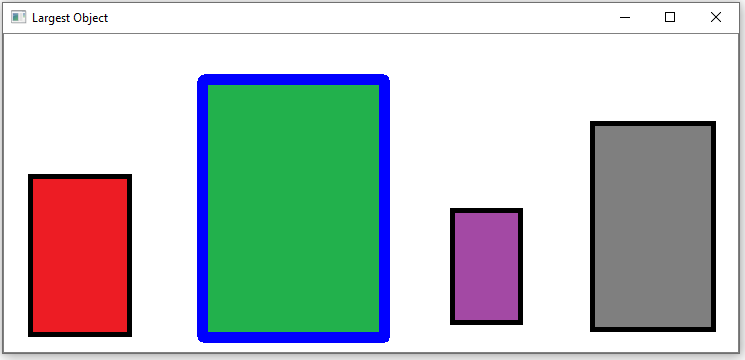﻿ How to Find the Largest or Smallest Object in an Image in Python using OpenCV ﻿# How to Find the Largest or Smallest Object in an Image in Python using OpenCVIn this article, we show how to find the largest or smallest object in an image in Python using the OpenCV module.

OpenCV is very dynamic in which we can first find all the objects (or contours) in an image using the cv2.findContours() function. We can then find the size of each object using the cv2.contourArea() function. We then organize these from largest to smallest or smallest or largest to get the largest or smallest object in our image.

So this is what we will do in this case.

In this example, we will be working with the following image shown below.As you can see in the above image, there are 4 rectangles, which we will say represents containers.

You can clearly see that the green rectangle is the largest object in this image.

The purple rectangle is the smallest object.

Below we'll see how this works in code.

### How to Find the Largest Object in an Image

We will first create code to find the largest object in this image and then we will draw an outline along the outer contour of the image.

This will produce the following image below.Let's now go over this code.

First, we import OpenCV using the line, import cv2

Next, we read in the image, which in this case is, Containers.png

We create the variable, original_image, to store the original image that will undergo modification throughout the code.

We create a grayscale version of the image and then find the Canny edges, which helps simplify the image for cvs.findContours() function. The cv2.findContours() function finds all external contours in our image, which finds the unique objects in an image.

We then create a function that finds all the areas of each of the contours in our image and stores this in a list in the variable, all_areas. This function returns this list.

We then sort this list from largest to smallest using the sorted() function. We store this sorted list into the variable, sorted_contours. We sort a list from largest to smallest by the attribute, reverse=True. To sort a list from smallest to largest by the attribute, reverse= False, or by specifying nothing in the reverse attribute, as reverse=False, is implied if not specified.

Now that the list is sorted from largest to smallest, the first item in the list is the largest item, which represents the largest object in our image. We get the largest item with the code, largest_item= sorted_contours

Lists always started with an index of 0. So the 0 index item is the first item and, thus, the largest object.

We then outline this largest object in blue with the drawContours() function.

### How to Find the Smallest Object in an Image

We will first create code to find the smallest object in this image and then we will draw an outline along the outer contour of the image.

This will produce the following image below.So everything is the same as before, except now we specify in the sorted() function that, reverse= False

Now the list is sorted from smallest to largest.

Therefore, now, the first item of the list is the smallest object.

And this is how we can find the largest or smallest object in an image by size in Python using OpenCV.

Related Resources